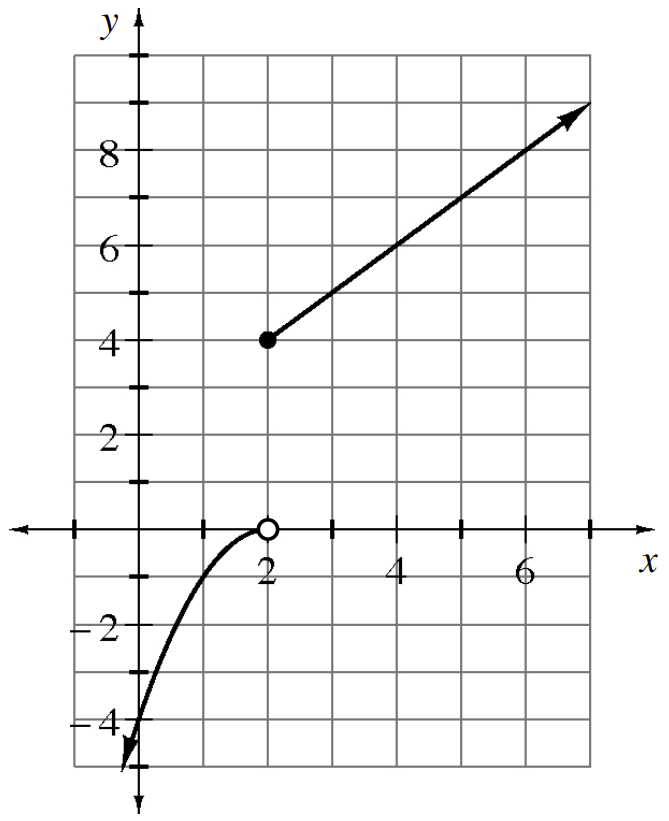Home > INT3 > Chapter 3 > Lesson 3.2.4 > Problem3-114

3-114.

Write a set of equations for the piecewise-defined function shown on the graph at right. Be sure to include the domain for each part of the function. Homework Help ✎

Look at the two pieces of the function. One is a downward-facing parabola moved $2$ to the right, and the other is a line.

$f(x)=\begin{cases}-(x-2)^2,x<2\\x+2,x\geq 2 \end{cases}$